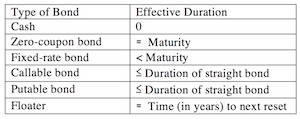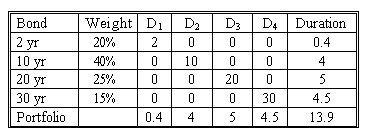### Seeing is believing!

Before you order, simply sign up for a free user account and in seconds you'll be experiencing the best in CFA exam preparation.

##### Subject 5. Duration
Bond duration measures the sensitivity of the bond's full price to changes in interest rates.

• Yield duration statistics measure the sensitivity of the bond's full price to the bond's own yield-to-maturity. They include the Macaulay duration, modified duration, money duration, and price value of a basis point. They can be used only for option-free bonds because they assume that a bond's expected cash flows do not change when the yield changes.
• Curve duration statistics measure the sensitivity of a bond's full price to the benchmark yield curve. An example is effective duration.

Effective duration measures interest rate risk in terms of a parallel shift of the benchmark yield curve.A pricing model can be used to estimate the price change resulting from a change in the benchmark yield curve instead of the bond's own yield-to-maturity. We use the binomial model to find both V- and V+. V- and V+ are adjusted to reflect any changes in the cash flows (due to embedded options) that result from the change in benchmark yield curve. An important assumption is that OAS stays constant when interest rates shift.

Steps:

• Calculate OAS, using the benchmark interest rate tree and the procedure described earlier.
• Increase all spot rates on the curve by y.
• Recalculate one-year rates on the tree.
• Add OAS from step 1 to all one-year rates at the nodes.
• Use the new rates adjusted for OAS in the backward induction process to calculate the new value of a bond, which is V+.
• Repeat steps 2 to 5, this time decreasing all initial spot rates by y to find V-.
• Calculate effective duration by substituting estimated V- and V+ into the formulas.

As yields decline, the duration of a callable bond shortens and approaches the duration to call. As yields rise, the duration lengthens and approaches the duration of a straight bond.

The opposite is true for a putable bond.

In either case the call/put option reduces the effective duration of the bond. The effective duration of a bond with embedded option(s) can never exceed that of the straight bond.One-Sided Durations

Because the prices of callable and putable bonds respond asymmetrically to upward and downward interest rate changes of the same magnitude, one-sided durations provide a better indication regarding the interest rate sensitivity of bonds with embedded options than (two-sided) effective duration.

Key-Rate Durations

It is important to distinguish interest rate risk from yield curve risk.

• The interest rate risk is the sensitivity of a bond to parallel shifts of the yield curve.
• The yield curve risk is a bond's sensitivity to changes in the shape of the yield curve.

Parallel shifts in the yield curve rarely occur. An analyst may want to measure the change in the bond's price by changing the spot rate for a particular key maturity and holding the spot rate for the other key maturities constant. The key rate duration is the sensitivity of the value of a bond to changes in a single spot rate, holding all other spot rates constant. There is a key rate duration for every point on the spot rate curve so there is a vector of durations representing each maturity on the spot rate curve.

In this approach, analysts calculate the sensitivity of the bond's price to changes in several key rates, such as 1-year, 2-year, 5-year and 10-year rates. An important assumption is that when one of the key rates is shocked, all other key rates remain constant. Several key rate durations result from this approach, one for each key rate.

• Every security in a portfolio has a set of key rate durations.
• Each key rate duration of a portfolio is the weighted average of key rate durations of individual securities.
• The sum of all key rate durations for a portfolio or an individual bond is equal to the modified duration of this portfolio or bond.

For an option-free bond:

• If it is trading at par, the maturity-matched par rate is the only rate that affects the bond's value.
• Key rate durations can be negative for maturity points that are shorter than the maturity of the bond if the bond has a very low or zero coupon.

For a bond with embedded options, the key rate durations depend on both the time to maturity and time to exercise.

Example

Suppose there are four key rates (2-years, 10 years, 20 years and 30 years). The duration of a zero-coupon bond is approximately the number of years to maturity. Therefore, the four key rate durations are 2, 10, 20 and 30. Consider the following portfolio:D1: key rate duration for the 2-year part of the curve. D2, D3, D4 ... All bonds are zero coupon bonds.

Let's calculate the 10-year duration of the portfolio. The duration of each zero-coupon bond equals its maturity. The 10-year key rate duration of the portfolio equals the product of 10-year zero's duration (which is 10) and the weight of this bond in the portfolio (40%). Therefore, the 10-year key rate duration of the portfolio is 10 x 0.4 = 4. To calculate the modified duration of the portfolio, we simply sum all its key rate durations 0.4 + 4 + 5 + 4.5 = 13.9.